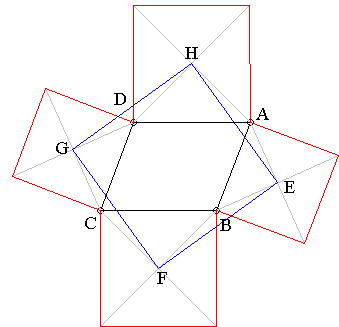# Thébault's Problem I What Is It? A Mathematical Droodle

### Thébault's Problem I

The applet suggests the following theorem (Victor Thébault, 1937):

On the sides of parallelogram $ABCD$ erect squares -- all either on the outside or the inside of the parallelogram. Their centers then form another square.### Proof 1

Consider the case of the squares erected on the outside of the parallelogram.

Triangles $AEH,$ $BFE,$ $CGF,$ and $DHG$ are obviously equal, so that the quadrilateral $EFGH$ is a rhombus. Further, $\angle DHG = \angle AHE,$ which implies $\angle GHE = \angle DHA = 90^{\circ}.$ Q.E.D.### Proof 2

Place the origin at the center of the parallelogram $ABCD,$ and let $u$ and $v$ be complex numbers associated with $D$ and $A.$ Then $DA$ is associated with $v - u,$ while $AB$ is corresponds to $-(v + u).$ Further,

$OH$ corresponds to $u + (v - u)/2 + i\cdot (v - u)/2 = (u + v)/2 + i\cdot (v - u)/2.$

Similarly we can compute $OE,$ $OF,$ $OG.$ For example,

$OE$ corresponds to $(v - u)/2 - i\cdot (u + v)/2.$

The difference of the two numbers corresponds to the side $EH$ of the quadrilateral EFGH and equals $u + iv.$ If the squares were constructed inwardly, $EH$ would correspond to $u - iv.$

Similarly, $v - iu$ corresponds to $GH.$ Since $v - iu = -i\cdot (u + iv),$ one is obtained from the other with the rotation through $90^{\circ}.$ In addition, $|v - i\cdot u| = |i\cdot (u + iv)| = |(u + iv)|.$ Therefore the quadrilateral $EFGH$ is a square.

Recall now the Parallelogram Law: for any two complex numbers $X$ and $Y,$

$|X + Y|^{2} + |X - Y|^{2} = 2(|X|^{2} + |Y|^{2}),$

from which we derive

\begin{align} |u + iv|^{2} + |u - iv|^{2} &= 2(|u|^{2} + |iv|^{2})\\ &= 2(|u|^{2} + |v|^{2})\\ &= |u - v|^{2} + |u + v|^{2}, \end{align}

which means that the sum of the areas of the two squares $EFGH$ (one constructed inwardly, the other outwardly) equals the sum of the areas of two distinct squares constructed on the sides of the parallelogram.

### Proof 3

Consider $\Delta ABD$ and the squares on sides $AD$ and $AB.$ As was shown elsewhere, the square whose diagonal coincides with EH has one of its vertices in the midpoint of $BD,$ i.e., $O,$ such that $\angle EOH = 90^{\circ}.$

### Proof 4

The tesselation of the plane into parallelograms and squares that serves to prove the Law of Cosines also provides an additional demonstration of Thebault's theorem.

### Proof 5

Grégoire Nicollier
University of Applied Sciences of Western Switzerland
Route du Rawyl 47, CH--1950 Sion, Switzerland
December 12, 2015

This is one of the series of spectral proofs.

Let $q=(1,\,i,\,-1,\,-i)$ be the positively oriented unit square of the complex plane. Any nontrivial parallelogram $Q$ centered at the origin of the complex plane can be expressed up to a spiral similarity as $Q=q+b\bar q$ for some $b=x+iy$ or $Q=\bar q$. We suppose $Q=q+b\bar q$. Let $T(Q)$ be the quadrilateral obtained by erecting isosceles right-angled right-hand ears on the sides of $Q$. The transformation $T$ is linear and deletes $\bar q$: thus $T(Q)=T(q+b\bar q)=T(q)=\sqrt2e^{i\pi/4}q$ is a square.

### References

1. R. Nelsen, Proofs Without Words II, MAA, 2000, p. 19

### Thébault's Problems

• Thébault's Problem I
• Thébault's Problem II
• Thébault's Problem III
• Y. Sawayama's Lemma
• Jack D'Aurizio Proof of Sawayama's Lemma
• Y. Sawayama's Theorem
• Thébault's Problem III, Proof (J.-L. Ayme)
• Circles Tangent to Circumcircle
• Thébault's Problem IV
• A Property of Right Trapezoids
• A Lemma on the Road to Sawayama
• Excircles Variant of Thébault's Problem III
• In the Spirit of Thebault I
• Dao's Variant of Thebault's First Problem
•